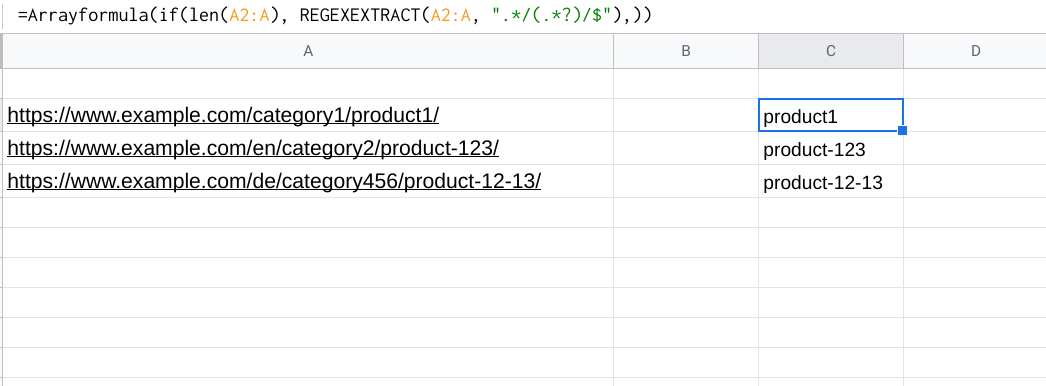# Các công thức thông dụng google sheet

Lọc tên miền

``````=INDEX(IFERROR(REGEXEXTRACT(A2;
"^(?:https?:\/\/)?(?:ftp:\/\/)?(?:www\.)?([^\/]+)")))
``````

Tìm giá trị trong cột

``````=IFERROR(ARRAYFORMULA(IF(SEARCH("/blog/",A2:A),"Blog Post","")),"")
``````
``````=SERPAPI_RESULT("q=coffee&engine=google&location=austin", "organic_results.0.title") Returns SerpApi result using any search engine and selector supported.
=SERPAPI_RANK("q=coffee&engine=google&location=austin", "yourwebsite.com") Returns rank of yourwebsite.com for Coffee in Austin.
``````

# Remove file extension from filename

## Summary

To remove a file extension from a file name, you can use a formula based on the LEFT and FIND functions. In the example shown, the formula in C5 is:

``````=LEFT(B5,FIND(".",B5)-1)
``````

## Generic formula

``````=LEFT(filename,FIND(".",filename)-1)
``````

## Explanation

The core of this formula is the LEFT function which simply extracts text from the file name, starting at the left, and ending at the character before the first period (“.”).

``````=LEFT(filename,characters)
``````

The FIND function is used to figure out how many characters to extract:

``````FIND(".",B5)-1
``````

Find returns the position of the first match (6 in the first example) from which 1 is subtracted. The result, 5, goes into LEFT like this:

``````=LEFT(B5,5)
``````

and the LEFT function returns the first five characters from the left: “Happy”.

Note: because this formula finds the first occurrence of “.”, it will remove all file extensions when there are are more than one.

Assuming the url’s in column A, try

``````=Arrayformula(if(len(A2:A), REGEXEXTRACT(A2:A, ".*/(.*?)/\$"),))
``````

and see if that works?